# Team:KU Leuven/Modeling/Internal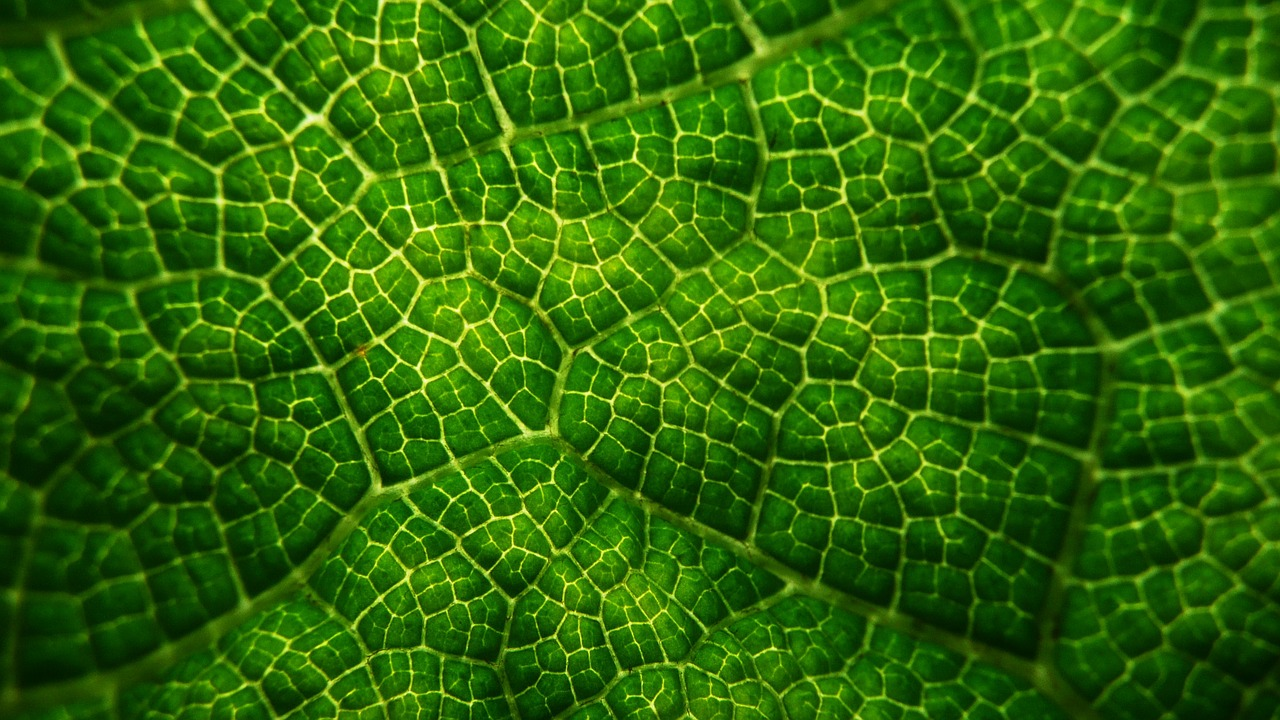## Introduction

We can think of many relevant questions when implementing a new circuit: how sensitive is the system, how much will it produce and will it affect the growth rate? As such, it is important to model the effect of the new circuits on the bacteria. This will be done in the Internal Model. We will use two approaches. First we will use a bottom-up approach. This involves building a detailed kinetic model with rate laws. We will use Simbiology and ODEs to study the sensitivity and dynamic processes inside the cell. Afterwards, a top-down model, Flux Balance Analysis (FBA), will be used to study the steady-state values for production flux and growth rate. This part is executed by the iGEM Team of Toulouse as part of a collaboration and can be found here

## Simbiology and ODEs

Our Simbiology model will be described in the next section. Simbiology is a toolbox from Matlab designed for the simulation of (bio)chemical reactions. It allows us to calculate systems of ODEs and to visualize the system in a diagram. It also has options to perform a sensitivity analysis, which allows us to study the effect of aspecified parameter. We will focus on the production of leucine, Ag43 and AHL in cell A and the changing behavior of cell B due to changing AHL concentration. In this perspective, we will make two models in Simbiology: one for cell A and one for cell B. First we will describe how we constructed the models and searched for parameters.

## Quest for parameters

We can divide the different processes going on in the cell in seven classes: transcription, translation, DNA binding, complexation and oligomerization, protein production kinetics, degradation and diffusion. We went on to search the necessary parameters and descriptions for each of these categories. To start constructing our model, we first have to pick a unit. We choose to use molecules as unit, because many constants are expressed in this unit and it allows us to drop the dillution terms connected to cell growth. We will also work with a deterministic model instead of a stochastic model.

The next step is to make some assumptions:

1. The effects of cell division can be neglected
2. The substrate pool can not be depleted and the concentration (or amount of molecules) of substrate in the cell is constant
3. The exterior of the cell contains no leucine at t=0 and is perfectly mixed
4. Extracellular diffusion of biomolecules happens much faster than the cell movement. Thus, diffusion is independent of cell movement and has a constant rate

## Transcription

Transcription is the first step of gene expression. It involves the binding of RNA polymerase to the promoter region of DNA and the formation of mRNA. The transcription rate is dependent on a number of conditions like the promoter strength and the used strain. Our system has both constitutive promoters, which are always active, and inducable promoters, which can be activated or repressed.

Maximum Transcription rate

First we will try to find the maximum transcription rate. The prediction of the transcription rate has been an important hold-back in the past, even though Polymerases per Second was introduced as a unit. This is the amount of Polymerases that passes through a given position in the DNA per time unit and is essentially the transcription rate at a particular location on the DNA . We can use this value as the transciption rate of the whole gene because it is the value of the slowest and thus rate-defining step, the binding of polymerase on the promoter. It remained difficult to measure in vivo but Kelly et al. have introduced a way to measure the activity of promoters using an in vivo standard, promoter J23101. This gave rise to a new unit: Relative activity of promoter (RPU)$=\frac{PoPS_{phi}}{PoPS_{J23101}}$ with $phi$ being the promoter of interest. Through the use of relative units, the variability due to equipment and conditions was drastically decreased. The PoPS of J23101 was found to be 0.03.

As our constitutive promoter, we used J23114. On the iGEM website we found that the whole J constitutive promoter family had been characterized. From these measurements we conclude that J23114 has a PoPS of 0.00429. The strength of the pLuxPR promoter has been measured relatively to pLacIQ by iGEM Tokyo_Tech 2010. Using these measurements, we calculate the PoPS of pLuxPR to be 0.00169 PoPS. Next we sought values for pCI. iGEM NYMU-Taipei 2009 measured the pLux promoter relative to pCI, which allows us to calculate the PoPS for pCI: $\frac{1}{0.43} {\cdot} 0.00169=0.0394$ PoPS. They also measured pPen from which we are able to calculate the PoPS: $0.3106 {\cdot} 0.0394=0.01224$.

These values all depend on measuring activities and since the strains, media and antibiotic markers won’t be completely the same, the real values will diverge from the values found with these simple calculations. Nevertheless, these values can give us an idea about the relative strength of the different promoters. We could measure the real values of mRNA concentration in our cells by executing a qPCR, but for now we will use the found values for our model. Another important parameter for transcription is the copy number in which the genes are present in the cell. The plasmid that incorporates the genes, has an ORI with a copy number between 100 and 300. In our model we will use a mean copy number of 200.

Inducable promoters

For inducable promoters the maximum transcription rate has to be multiplied with a factor to integrate the effect of the transcription factors. Our system includes two types of DNA binding proteins: repressors and activators. These proteins bind certain regions in the promoter region of the DNA and can either repress or activate the promoter activity, affecting transcription rate. The repressor proteins used in our system are the cI protein of the lambda phage and the PenI protein. The activator protein is LuxR, which has to be activated with AHL. The proteins first need to form dimers before they are able to bind their target DNA.

DNA binding will be simulated using the Hill function : ${\theta} = \frac{{[L]}^n}{{K_d}^n + [L]^n} = \frac{1}{1+(\frac{K_{d}}{[L]})^{n}}$. $\theta$ is the amount of DNA bound by the protein, $L$ is the amount of protein, $K_d$ is the dissociation constant and $n$ is the Hill coefficient . This Hill function has values between 0 and 1 and acts like an ON/OFF switch.
For the repressors, we are interested in how much of the DNA is still unbound and active: $\frac{1}{1 + ({\frac{[Repressor]}{K_{d}}})^{n}}$
For the activators, we are interested in how much of the DNA is bound and active: $\frac{1}{1+(\frac{K_{d}}{[Activator]})^{n}}$
The Hill coefficient gives an idea about the strength about the interaction between the DNA and the protein and is sometimes used to estimate the amount of proteins that bind the DNA, but caution is needed in using it. Indeed, the Hill function has known shortcomings, but it is still very useful because of its simplicity .

The constants needed for our model are $K_d$ and $n$. :

#### Table 1: The constants of the Hill function for cI, LuxR and PenI

Protein Kd (molecules) n Source
cI 20 2 2009 iGEM Aberdeen and Wang et al. (2009) 
LuxR/AHL 3.7 1 2007 iGEM ETH Zurich and Basu et al. (2005) 
PenI 17 2 Wittman et al. (1993) 

${K_d}$ is the value which corresponds with the amount of repressor or activator necessary to repress or activate half of the promoters. Since our copynumber is 200, we multiply the Hill constants with 200 because this higher value seems more logical to us.

Leakiness

A last important value in transcription is the leakiness of the promoter. A shutdown promoter still has a very small transcription which is called the leakiness of the promoter. This value should be as low as possible, because there should be a big difference between ON and OFF promoters. The effect of leakiness of promoters is something that should be checked.

iGEM 2012 Upssala measured the leakiness of the pLuxPR promoter. Without AHL and LuxR, the pLuxPR was partially active and had a RPU of 10. This means that the leaking promoter has a PoPS of $\frac{10}{390} {\cdot} 0.040 {\cdot} 0.03 = 3.077 {\cdot} 10^{-5} PoPS$. We can use this value to estimate the leakiness of the other promoters.

## Translation

The next step in gene expression is the translation of the synthesized mRNA to a protein. This involves the binding of a ribosome complex to the RBS site of the mRNA, followed by elongation and termination. The initiation step is the rate-limiting step of the three. The translation rate is thus tied to the thermodynamics of RBS-ribosome interaction, but other sequences (5’-UTR, Shine-Delgarno,repeats, internal startcodons) and possible secondary structures (hairpins) also play a role . Salis et al have developed an algorithm to predict the translation initiation rate based upon thermodynamic calculations. This algorithm is used in the Ribosome Binding Site Calculator9. We used this calculator to predict the translation rates of our mRNAs.

The results of the calculator are shown in table 2. The program gives us results in au (arbitrary units). Since we know the translation rate of LuxI and LuxR, we can use these as a standard to calculate the other translation rates since the used scale is proportional. LuxI and LuxR have almost the same output from the calculator which corresponds with the paper where they also have the same translation rate. Our values are also normal values since values between 1 and 100 000 are possible. We did get warnings about the accuracy of the prediction (NEQ: not at equilibrium) which happens when mRNA may not fold quickly to its equilibrium state.

#### Table 2: The translation rates for the different proteins as found by the RBS calculator using LuxR and LuxI as standard

Protein Translation rate (a.u.) Translation rate (1/s)
LuxI 261.13 0.016
LuxR 279.61 0.016
cI857 5695.79 0.35
Transaminase B 2195.1 0.13
Ag43-YFP 33.22 0.0020
PenI 6696.73 0.41
RFP 2604.51 0.16
CheZ-GFP 396.95 0.024

For Transaminase B we also need to add a production term because it is a protein which is also naturally occurring in E. coli. We estimate a value of 0.0033 for this constitutive production rate.

## Complexation and oligomerization kinetics

Before the proteins can bind the promoter region, they first have to form complexes. cI and PenI form homodimers, while LuxR first forms a heterodimer with AHL and afterwards reacts to form a homodimer with another LuxR/AHL molecule. This next part will describe the kinetics of such complexation. The kinetics will be modelled by simple mass action rates. We only need the parameters of LuxR and cI, because the Hill function found for PenI was already adapted for the protein in monomer form.

#### Table 3: Dissociation and association rates for the oligomerization of the transcription factors used in our circuit

Biomolecule Oligomerization rate Source
LuxR/AHL association $1{\cdot}10^{-5}$ 1/(molecule*s) Goryachev et al. (2005) 
LuxR/AHL dissociation 0.00333 1/s Goryachev et al. (2005) 
LuxRdimer association $1{\cdot}10^{-5}$ 1/(molecule*s) Goryachev et al. (2005) 
LuxRdimer dissociation 0.01 1/s Goryachev et al. (2005) 
cI dimer association 0.00147 1/(molecule*s) Samiee et al. (2005) 
cI dimer dissociation 0.01 1/s Samiee et al. (2005) 

## Protein production kinetics

Our models comprises two product forming enzymes: LuxI and Transaminase B. LuxI is the enzyme responsible for AHL production and Transaminase B is responsible for leucine formation. Therefore, we need to describe the following reactions:
$$\text{acylACP} + \text{SAM} \rightarrow \text{ACP} + \text{MTA} + \text{AHL}$$ $$\text{glutamate} + \text{aKIC} \rightarrow \text{aKG} + \text{Leucine}$$
For LuxI kinetics Schaefer et al. (1996) found a value of 1 molecule/minute as Vmax. Since we assume that LuxI is fully saturated with substrate, we take this value as the synthesis rate of AHL. 

Transaminase B synthesizes leucine from aKIC and glutamate. In this reaction glutamate is converted to aKG. The reaction kinetics is of the Ping-Pong Bi-Bi form. This model describes a mechanism in which the binding of substrates and release of products is ordered. The enzyme shuttles between a free and a substrate-modified intermediate state. 

## Ping-Pong Bi-Bi equations

\begin{align}\frac{{\large d} Transaminase B}{d t} = & \beta_{TB} {\cdot} {m_{ilvE}} - kf_1 {\cdot} {Transaminase B} {\cdot}{Glutamate} + kf_{-1}{\cdot}{[TB-GLU]} \\\\&- kr_1 {\cdot}{Leucine_{in}}{\cdot}{Transaminase B} + kr_{-1}{\cdot}{TB-Leu} + kcat2{\cdot}{[{TBNH}_2-aKIC]} \\\\&+ kcat4{\cdot}{[{TBNH}_2-aKG]} + k_{production} - d_{TB} {\cdot} {Transaminase B}\end{align} $$\frac{{\large d}{[TB-GLU]}}{d t}= -{kcat1}{\cdot}{[TB-GLU]} + {kf}_{1}{\cdot}{TB}{\cdot}{Glu} - {kf}_{-1}{\cdot}{[TB-GLU]}$$ \begin{align} \frac{{\large d}{[{TBNH}_2]}}{d t}= & {kcat1}{\cdot}{[TB-GLU]} + {kcat3}{\cdot}{[TB-Leu]}- {kf}_{2}{\cdot}{{TBNH}_2}{\cdot}{aKIC} + {kf}_{-2}{\cdot}{[{TBNH}_2-aKIC]} \\\\ & - {kr}_2{\cdot}{{TBNH}_2}{\cdot}{aKG} +{kr}_{2}{\cdot}{[{TBNH}_2-aKG]} \end{align} $$\frac{{\large d}{[{TBNH}_2-aKIC]}}{d t}= -{kcat2}{\cdot}{[{TBNH}_2-aKIC]} + {kf}_{2}{\cdot}{{TBNH}_2}{\cdot}{aKIC} - {kf}_{-2}{\cdot}{[{TBNH}_2-aKIC]}$$ $$\frac{{\large d}{[TB-Leu]}}{d t}= {kr}_1{\cdot}{Leucine}_{in}{\cdot}{[TB-GLU]} - {kr}_{-1}{\cdot}{[TB-Leu]} - {kcat3}{\cdot}{[TB-Leu]}$$ $$\frac{{\large d}{[{TBNH}_2-aKG]}}{d t}= {kr}_2{\cdot}{{TBNH}_2}{\cdot}{aKG} - {kr}_{2}{\cdot}{[{TBNH}_2-aKG]} - {kcat4}{\cdot}{[{TBNH}_2-aKG]}$$

Yang et al. (2005) studied these reactions and found a method to estimate the constants: the Lambda approximation . Their constants of this reversible Ping-Pong Bi-Bi reactions are put in the next table :

#### Table 4: Constants of the Ping-Pong Bi-Bi reactions using Transaminase B as found by Yang et al. (2005) 

Constant Value
${kf}_{1}$ 0.0098
${kf}_{-1}$ 3300
${kf}_{2}$ 0.0882
${kf}_{-2}$ 5940
${kr}_{1}$ 0.0031184
${kr}_{-1}$ 4620
${kr}_{2}$ 0.0041161
${kr}_{-2}$ 3465
$kcat1$ 33.33
$kcat2$ 60
$kcat3$ 46.67
$kcat4$ 35

Proteins, mRNA and other metabolites have a turnover rate. They are degraded over time. The degradation rate will be described with first order kinetics where d represents the degradation constant. Not every molecule has the same degradation rate since some molecules are more stable than others. We can influence the stability of the molecules. For example, in cell B it is important that there is a fast switch between conditions and a fast turnover of CheZ and RFP is necessary. This is why we add a LVA-tag to these proteins. This tag destabilizes the protein and makes them degradade faster. For Transaminase B we choose a very high degradation rate, because we did not include degradation terms for the Transaminase B bound to substrate. The degradation rates used in the model are put in the next table:

#### Table 5: Degradation rates for the different biomolecules used in our circuit

LuxI $5 \cdot 10^{-5}$ Goryachev et al. (2005) 
luxI-mRNA 0.006 Goryachev et al. (2005) 
LuxR $2 \cdot 10^{-4}$ Goryachev et al. (2005) 
luxR-mRNA 0.006 Goryachev et al. (2005) 
cI857 0.00288 iGEM Aberdeen 2009
cI-mRNA 0.002265 Bernstein et al. (2002) 
Transaminase B 4.5 Estimated
ilvE-mRNA 0.00304 Bernstein et al. (2002) 
Ag43-YFP $2.84{\cdot}10^{-5}$ Bernstein et al. (2002) 
Adhesine mRNA 0.00186 Bernstein et al. (2002) 
PenI 0.00333 Estimated
PenI mRNA 0.002265 Bernstein et al. (2002) 
RFP-LVA 0.0002814 Andersen et al. (1998) and iGEM KULeuven 2008
RFP mRNA 0.066 Bernstein et al. (2002) 
CheZ-GFP-LVA 0.0002814 Andersen et al. (1998) 
CheZ-GFP mRNA 0.00333 Estimated
AHL $1.667 \cdot 10^{-4}$ Basu et al. (2005) 
AHL external $1.155 \cdot 10^{-5}$ Horswill et al. (2007) 
leucine 0.0167 Estimated
leucine external 0.000333 Estimated

## Diffusion

Our model has two types of diffusion. Diffusion over the cell membrane from the inside of the cell to the outside and diffusion in the external medium. The diffusion over the cell membrane is more complicated because proteins play a role in it and the membrane is not equally permeable for every molecule. For diffusion we need to change our unit because diffusion is usually used with concentration as unit. This is why we added a correction term for the volume of the cell and the external volume.

We assume that the volume of the cell has a shape of a cilinder. For this simplified volume Goryachev et al. found a value of $5.65 {\cdot} 10^{-16}l$ (radius=0.3µm, length=2µm). We can take this volume as a constant, since cell growth is very small compared to the diffusion. The outside compartment will be modeled as half a sphere with the cell as center and a radius equal to $\sqrt{2{\cdot}D{\cdot}t} + r_0$. We only take half a sphere because there is no upward diffusion. For the initial value of the outside compartment we take a volume (and thus $r_0$ slightly bigger than the cell volume (radius). We choose a value of $5.7 {\cdot} 10^{-16}$l which accords to a radius of $5.128{\cdot}10^{-6} dm$. Because there is also already a volume taken by the cell, the total initial outside compartment has an initial value of $5{\cdot}10^{-18} l$. The volume will increase per time step with $\frac{2{\cdot}\pi{\cdot}D}{\sqrt{2{\cdot}D{\cdot}t}}{\cdot}{(\sqrt{2{\cdot}D{\cdot}t} + r_0)^2}$

With this approximation we get more logical results. Now we can calculate the diffusivity of AHL and leucine. Goryachev et al. used this formule $$D_{AHL,in}=\frac{P{\cdot}{S_{cell}}}{V_{cell}}$$ with P equal to $(1-5){\cdot}10^{-6} cm^2/s$ and $S_{cell}=4.34{\cdot}10^{-8} cm^2$. This formula gives us $D_{AHL,in}$=0.08-0.4 1/s. We take the diffusion rate for AHL equal to 0.23 1/s. For leucine we make a difference between inward and outward diffusion. Since leucine is an amino acid, a cell does not want to lose its leucine but it will want to take leucine in. Inward diffusion is thus facilitated by transporters, so we choose this value to be the largest. The inward diffusion rate is estimated at 0.05 1/s and the outward diffusion 0.0005 1/s.

#### Table 6: Diffusion rates of the molecules in our circuit

Biomolecule Diffusion rate (1/s) Source
AHL IN and OUT 0.23 1/s Goryachev et al. (2005) 
leucine IN 0.05 1/s Estimated
leucine OUT 0.0005 1/s Estimated
AHL extracellular $7.1{\cdot}10^{-9}$ dm²/s Goryachev et al. (2005) 
leucine extracellular $7.3{\cdot}10^{-8}{\cdot}0.09$ dm²/s Estimated

## System

After this extensive literature search, we can finally set up our complete system of ODEs for every cell.

## Cell A

The designed circuit in cell A is under control of a temperature sensitive cI repressor. Upon raising the temperature, cI will dissociate from the promoter and the circuit is activated. This leads to the initiation of the production of LuxR and LuxI. LuxI will consecutively produce AHL, which binds with LuxR. The newly formed complex will then activate the production of leucine and Ag43. Leucine and AHL are also able to diffuse out of the cell into the medium. Ag43 is the adhesine which aids the aggregation of cells A, while leucine and AHL are necessary to repel cells B.

We can extract the following ODE's from this circuit:

## Cell A equations

Symbols:${}$ ${\alpha}$: transcription term, ${\beta}$: translation term, $d$: degradation term,
$D$: diffusion term, ${ K_d}$: dissociation constant, n: Hill coefficient, L: leak term

$$\frac{{\large d} m_{cI}}{d t} = \alpha_1 {\cdot} cI_{gene} - d_{mCI} {\cdot} m_{cI}$$ \begin{align} \frac{{\large d}{cI}}{d t} = \beta_{cI} {\cdot} {m_{cI}} -2 {\cdot} {k_{cI,dim}} {\cdot} {cI}^2 + 2 {\cdot} {k_{-cI,dim}}{\cdot} {[cI]_2} - d_{cI} {\cdot} {cI} \end{align} $$\frac{{\large d}{[cI]_2}}{d t}= k_{cI,dim} {\cdot} {cI}^2 - {k_{-cI,dim}}{\cdot} {[cI]_2}$$ $$\frac{{\large d} m_{LuxI}}{d t} = (L_{lambda} + {\frac{\alpha_{lambda}}{1 + ({\frac{[cI]_2}{K_{d1}}})^{n_{cI}}}}) {\cdot} LuxI_{gene} - d_{mLuxI} {\cdot} m_{LuxI}$$ $$\frac{{\large d} m_{LuxR}}{d t} = (L_{lambda} + {\frac{\alpha_{lambda}}{1 + ({\frac{[cI]_2}{K_{d1}}})^{n_{cI}}}}) {\cdot} LuxR_{gene} - d_{mLuxR} {\cdot} m_{LuxR}$$ $$\frac{{\large d} LuxI}{d t} = \beta_{LuxI} {\cdot} {m_{LuxI}} - d_{LuxI} {\cdot}{LuxI}$$ $$\frac{{\large d} LuxR}{d t} = \beta_{LuxR} {\cdot} {m_{LuxR}} -k_{lux,as} {\cdot}{LuxR}{\cdot}{AHL_{in}} + k_{lux,dis}{\cdot}{[LuxR/AHL]} - d_{LuxR} {\cdot}{LuxR}$$ \begin{align}\frac{{\large d} AHL_{in}}{d t} = &{k_{luxI}} {\cdot} {luxI} - k_{lux,as} {\cdot}{luxR}{\cdot}{AHL_{in}} + k_{lux,dis}{\cdot}{[luxR/AHL]} \\\\& + ( {D_{IN,AHL}} {\cdot} {AHL_{out}} {\cdot}\frac{{V_{cell}}}{V_{external,AHL}} - {D_{OUT,AHL}} {\cdot} {AHL_{in}} ) - d_{AHL,in} {\cdot} {AHL_{in}} \end{align} \begin{align} \frac{{\large d} AHL_{out}}{d t} =& ( {D_{OUT,AHL}} {\cdot} {AHL_{in}} {\cdot}\frac{V_{external,AHL}}{{V_{cell}}} \\\\&-{D_{IN,AHL}}{\cdot}{AHL_{out}} ) -d_{AHL,out}{\cdot}{AHL_{out}} \end{align} $$\frac{{\large d} [luxR/AHL]}{d t} = k_{lux,as} {\cdot}{luxR}{\cdot}{AHL_{in}} - k_{lux,dis}{\cdot}{[luxR/AHL]} - 2 {\cdot} k_{lux,dim} {\cdot}{[luxR/AHL]^2} + 2 {\cdot}{k_{-lux,dim}}{\cdot}{[luxR/AHL]_{2}}$$ $$\frac{{\large d} [luxR/AHL]_{2}}{d t} = k_{lux,dim} {\cdot}{[luxR/AHL]^2} - k_{- lux,dim} {[luxR/AHL]}$$ $$\frac{{\large d} m_{ilvE}}{d t} = (L_{lux} + \frac{\alpha_{lux}}{1+(\frac{K_{d2}}{[luxR/AHL]_{2}})^{n_{lux}}} ) {\cdot} ilvE_{gene} - d_{milvE} {\cdot} {m_{ilvE}}$$ $$\frac{{\large d} m_{Ag43}}{d t} = (L_{lux} + \frac{\alpha_{lux}}{1+(\frac{K_{d2}}{luxR/AHL]_{2}})^{n_{lux}}} ) {\cdot} Ag43_{gene} - d_{mAg43} {\cdot} {m_{Ag43}}$$ $$\frac{{\large d} Ag43}{d t} = \beta_{Ag43} {\cdot} {m_{Ag43}} - d_{Ag43} {\cdot} {Ag43}$$ \begin{align}\frac{{\large d} Transaminase B}{d t} = & \beta_{TB} {\cdot} {m_{ilvE}} - kf_1 {\cdot} {Transaminase B} {\cdot}{Glutamate} + kf_{-1}{\cdot}{[TB-GLU]} \\\\&- kr_1 {\cdot}{Leucine_{in}}{\cdot}{Transaminase B} + kr_{-1}{\cdot}{TB-Leu} + kcat2{\cdot}{[{TBNH}_2-aKIC]} \\\\&+ kcat4{\cdot}{[{TBNH}_2-aKG]} + k_{production} - d_{TB} {\cdot} {Transaminase B}\end{align} $$\frac{{\large d}{[TB-GLU]}}{d t}= -{kcat1}{\cdot}{[TB-GLU]} + {kf}_{1}{\cdot}{TB}{\cdot}{Glu} - {kf}_{-1}{\cdot}{[TB-GLU]}$$ \begin{align} \frac{{\large d}{[{TBNH}_2]}}{d t}= & {kcat1}{\cdot}{[TB-GLU]} + {kcat3}{\cdot}{[TB-Leu]}- {kf}_{2}{\cdot}{{TBNH}_2}{\cdot}{aKIC} + {kf}_{-2}{\cdot}{[{TBNH}_2-aKIC]} \\\\ & - {kr}_2{\cdot}{{TBNH}_2}{\cdot}{aKG} +{kr}_{2}{\cdot}{[{TBNH}_2-aKG]} \end{align} $$\frac{{\large d}{[{TBNH}_2-aKIC]}}{d t}= -{kcat2}{\cdot}{[{TBNH}_2-aKIC]} + {kf}_{2}{\cdot}{{TBNH}_2}{\cdot}{aKIC} - {kf}_{-2}{\cdot}{[{TBNH}_2-aKIC]}$$ $$\frac{{\large d}{[TB-Leu]}}{d t}= {kr}_1{\cdot}{Leucine}_{in}{\cdot}{[TB-GLU]} - {kr}_{-1}{\cdot}{[TB-Leu]} - {kcat3}{\cdot}{[TB-Leu]}$$ $$\frac{{\large d}{[{TBNH}_2-aKG]}}{d t}= {kr}_2{\cdot}{{TBNH}_2}{\cdot}{aKG} - {kr}_{2}{\cdot}{[{TBNH}_2-aKG]} - {kcat4}{\cdot}{[{TBNH}_2-aKG]}$$ \begin{align}\frac{{\large d}{Leucine_{in}}}{d t}=&{kcat2}{\cdot}{[{TBNH}_2-aKIC]} - {kr}_1 {\cdot}{Leucine_{in}}{\cdot}{Transaminase B} + {kr}_{-1}{\cdot}{[TB-Leu]} \\\\&- d_{Leu}{\cdot}{Leucine_{in}} - {D_{OUT,Leu}}{\cdot}{Leucine_{in}} + {D_{IN,Leu}}{\cdot}{Leucine_{out}}{\cdot}\frac{{V_{cell}}}{V_{external,leu}} \end{align} $$\frac{{\large d} Leucine_{out}}{d t} = (D_{OUT,Leu} {\cdot}{leucine_{in}}{\cdot}\frac{V_{external,leu}}{{V_{cell}}} - D_{IN,Leu} {\cdot}{leucine_{out}}) - d_{Leu,out} {\cdot} {Leucine_{out}}$$ $$\frac{{\large d} V_{external,AHL}}{d t} = \frac{2{\cdot}\pi{\cdot}D_{AHL}}{\sqrt{2{\cdot}D{\cdot}t}}{\cdot}{(\sqrt{2{\cdot}D{\cdot}t} + r_0)^2}$$ $$\frac{{\large d} V_{external,Leu}}{d t} = \frac{2{\cdot}\pi{\cdot}D_{Leu}}{\sqrt{2{\cdot}D{\cdot}t}}{\cdot}{(\sqrt{2{\cdot}D{\cdot}t} + r_0)^2}$$

We visualize these ODE's in the Simbiology Toolbox which results in the following diagram: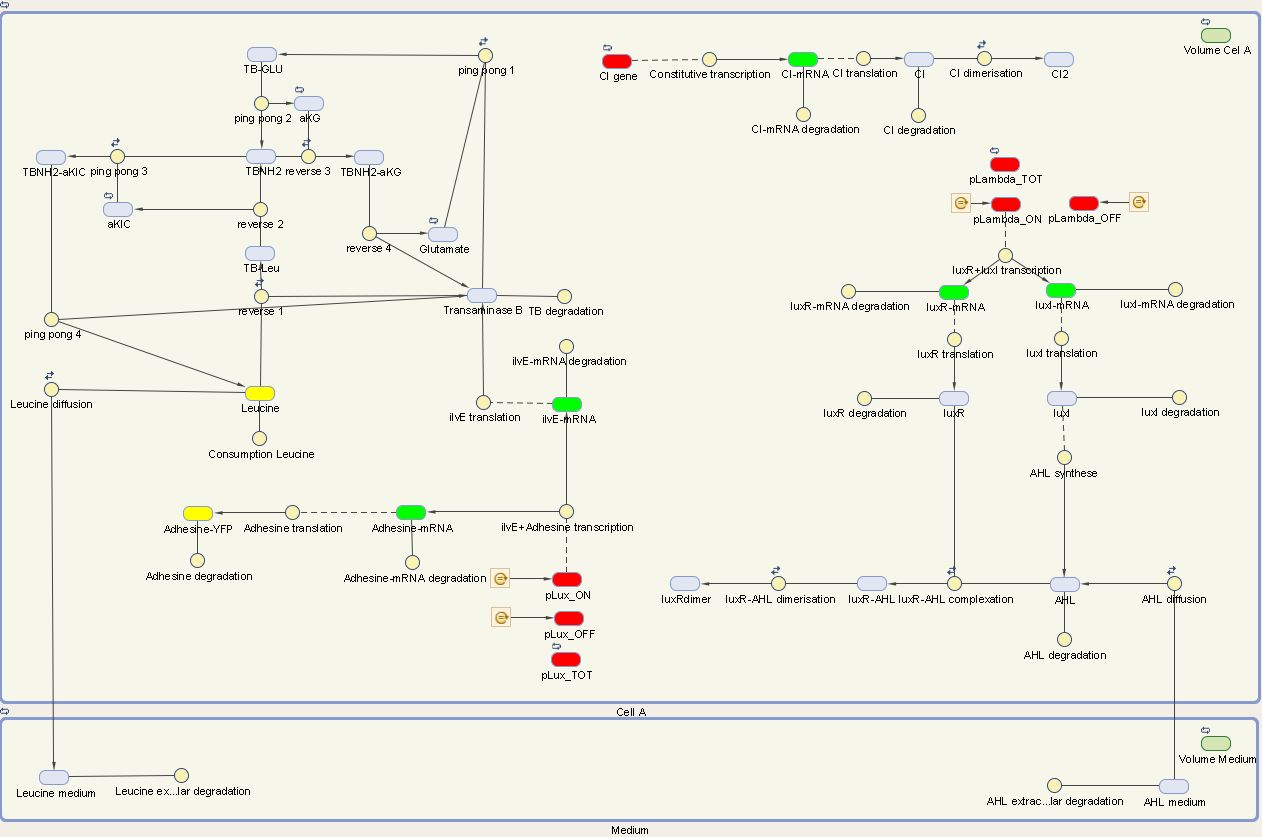## Cell B

The system of cell B is also under control of the cI repressor and is activated similar as cell A. The activation by the temperature raise, leads to the production of LuxR. AHL of the medium can diffuse into the cell, binding LuxR and activating the next component of the circuit. This leads to the production of CheZ and PenI. CheZ is the protein responsible for cells to make a directed movement, governed by the repellent leucine. PenI is a repressor which will shut down the last part of the circuit which was responsible for the production of RFP.

We can extract the following ODEs for cell B from this sytem:

## Cell B equations

Symbols: ${\alpha}$:transcription term, ${\beta}$:translation term, $d$:degradation term,
$D$:diffusion term, ${ K_d}$:dissociation constant, n:Hill coefficient, L:leak term

$$\frac{{\large d} m_{cI}}{d t} = \alpha_1 {\cdot} cI_{gene} - d_1 {\cdot} m_{cI}$$ $$\frac{{\large d}{cI}}{d t} = \beta_1 {\cdot} {cI} -2 {\cdot} {k_{cI,dim}} {\cdot} {cI}^2 + 2 {\cdot}{k_{-cI,dim}}{\cdot} {[cI]_2} - d_{cI} {\cdot} {cI}$$

$$\frac{{\large d}{[cI]_2}}{d t}= k_{cI,dim} {\cdot} {cI}^2 - {k_{-cI,dim}}{\cdot} {[cI]_2}$$ $$\frac{{\large d} m_{luxR}}{d t} = (L_{lambda} + {\frac{\alpha_{lambda}}{1 + ({\frac{cI}{K_{d1}}})^{n_{cI}}}}) {\cdot} luxR_{gene} - d_{mluxR} {\cdot} m_{luxR}$$ $$\frac{{\large d} luxR}{d t} = \beta_{luxR} {\cdot} {m_{luxR}} - d_{luxR} {\cdot}{luxR}$$ $$\frac{{\large d} AHL_{in}}{d t} = ( {D_{IN,AHL}} {\cdot} {AHL_{out}} - {D_{OUT,AHL}} {\cdot} {AHL_{in}} ) - k_{lux,as}{\cdot}{luxR}{\cdot}{AHL_{in}} + k_{lux,dis}{\cdot}{[luxR/AHL]} - d_{AHL,in} {\cdot} {AHL_{in}}$$ $$\frac{{\large d} AHL_{out}}{d t} = ( {D_{OUT,AHL}} {\cdot} {AHL_{in}} - {D_{IN,AHL}}{\cdot}{AHL_{out}} ) -d_{AHL,out}{\cdot}{AHL_{out}}$$ $$\frac{{\large d} [luxR/AHL]}{d t} = k_{lux,as} {\cdot}{luxR}{\cdot}{AHL_{in}} - k_{lux,dis}{\cdot}{[luxR/AHL]} - 2 {\cdot} k_{lux,dim} {\cdot}{[luxR/AHL]^2} + 2 {\cdot}{[luxR/AHL]_{2}}$$ $$\frac{{\large d} [luxR/AHL]_{2}}{d t} = k_{lux,dim} {\cdot}{[luxR/AHL]^2} - k_{- lux,dim} {[luxR/AHL]}$$ $$\frac{{\large d} m_{CheZ}}{d t} = (L_{lux} + \frac{\alpha_{lux}}{1+(\frac{K_{d2}}{[luxR/AHL]_{2}})^{n_{lux}}} ) {\cdot} CheZ_{gene} - d_{mCheZ} {\cdot} {m_{CheZ}}$$ $$\frac{{\large d} m_{PenI}}{d t} = (L_{lux} + \frac{\alpha_{lux}}{1+(\frac{K_{d2}}{luxR/AHL]_{2}})^{n_{lux}}} ) {\cdot} PenI_{gene} - d_{mPenI} {\cdot} {m_{PenI}}$$ $$\frac{{\large d} CheZ}{d t} = \beta_{CheZ} {\cdot} {m_{CheZ}} - d_{CheZ} {\cdot} {CheZ}$$ $$\frac{{\large d} PenI}{d t} = \beta_{PenI} {\cdot} {m_{PenI}} - d_{PenI} {\cdot} {PenI}$$ $$\frac{{\large d} m_{RFP}}{d t} = (L_{Pen} + {\frac{\alpha_{Pen}}{1 + ({\frac{PenI}{K_{d3}}})^{n_{Pen}}}}) {\cdot} RFP_{gene} - d_{mRFP} {\cdot} m_{RFP}$$ $$\frac{{\large d} RFP}{d t} = \beta_{RFP} {\cdot}{m_{RFP}} - d_{RFP} {\cdot}{RFP}$$

We visualize these ODE's in the Simbiology toolbox. This gives us the following diagrams: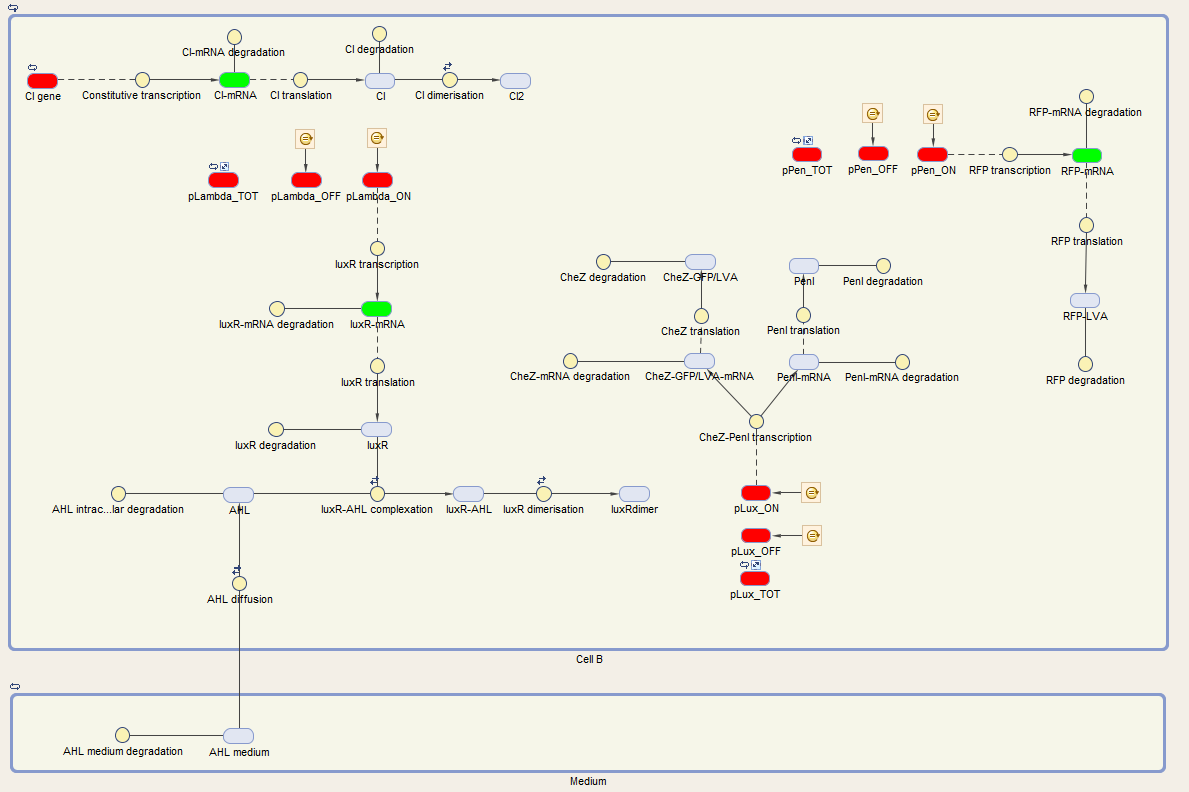#### Figure 2: The internal network of cell A visualized by Simbiology## Results

The simulations will be made using ode15s, a solver for stiff systems. We also need to define initial values. We take for the external volume $5{\cdot}10^{-18}l$ as initial value, and for cI-mRNA, cI and $cI_2$ 300,2000, $6{\cdot}10^5$ molecules respectively.

For cell A we made a simulation with cell A in the ON and OFF mode as visuable in figure 3. When cell A is in the OFF state, the whole designed circuit is in OFF mode. This means that the cI repressor is succesful in repressing the design. If the degradation rate of cI is raised to simulate the temperature rising, we see that all the components of the system show a big increase. For LuxR this increase is only temporary, but this is also explainable. Since LuxI keeps on producing AHL which binds LuxR to form a complex. Indeed all the LuxR reacts to form the complex. Some values seem really high (for example the AHL,out en leucine,extracellular values, but they are also in the biggest volume so the concentration is not that high). We also assumed that there is a substrate pool without limiting, which is of course not the real situation. The most important conclusion is that with values backed up by literature, our system qualitatively still shows the desired behavior. We can also ignore noise effects because our model works with a big amount of transcription factors, so small changes will only have a limited effect.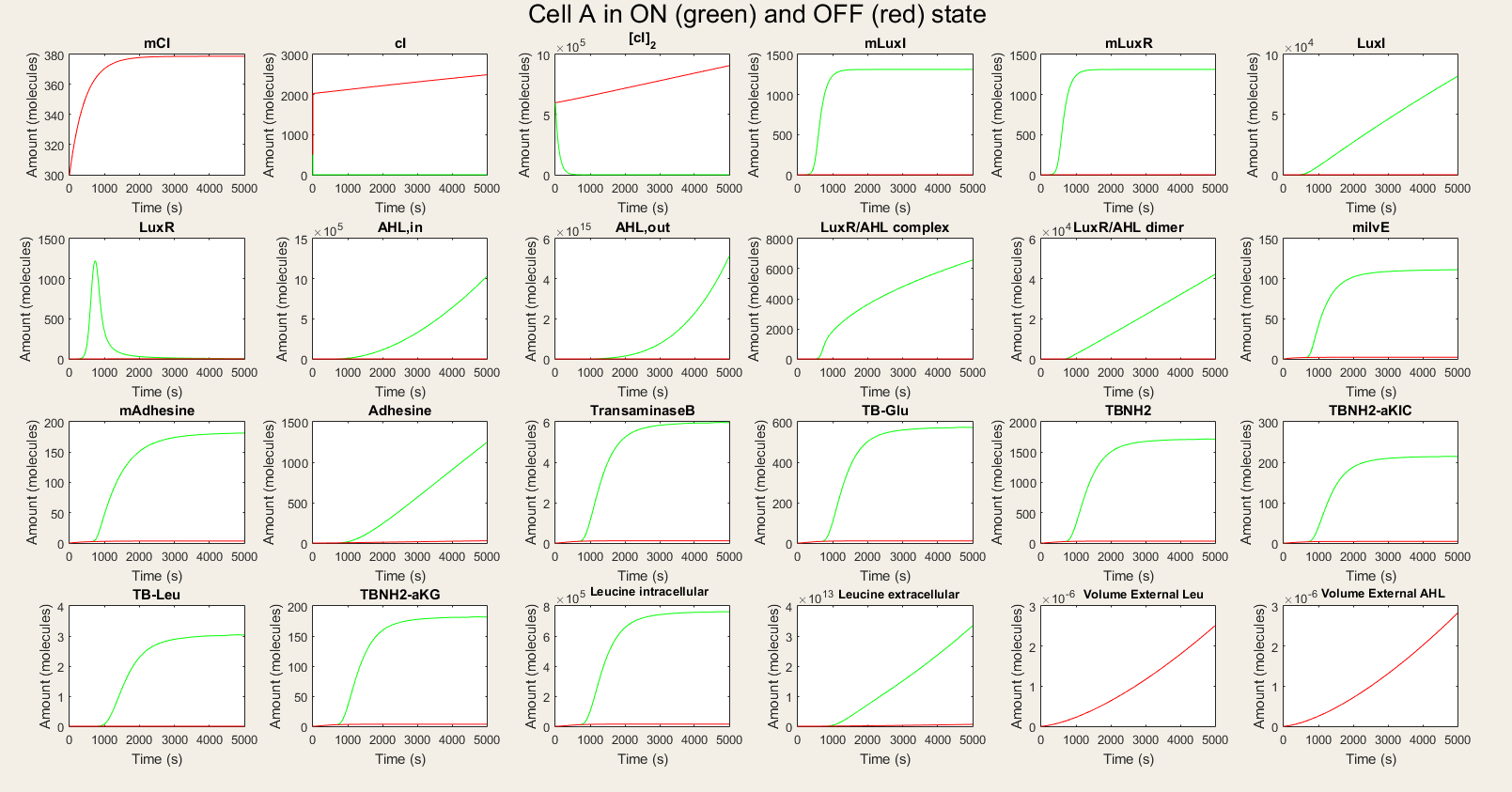#### Figure 3: Simulation of all processes in Cell A in ON and OFF state

Afterwards, we made the simulations for cell B. In the OFF state simulations, there is not a big difference between the red and green lines. We do see a very small rise in LuxR but it is not significant. We see a fast equilibration between the external AHL and internal AHL and no drop since there is no LuxR to react with the AHL. The only proteins that are available in high amounts are PenI and RFP. The high amount of PenI was not predicted in our design, but it does not affect the amount of RFP.

In the ON simulations we see a big difference between the red and green lines. When there is AHL available, the production of CheZ and PenI is much higher and the production of RFP much lower. This is expected the behavior. Therefore we conclude that our system is still qualitatively showing the desired behavior.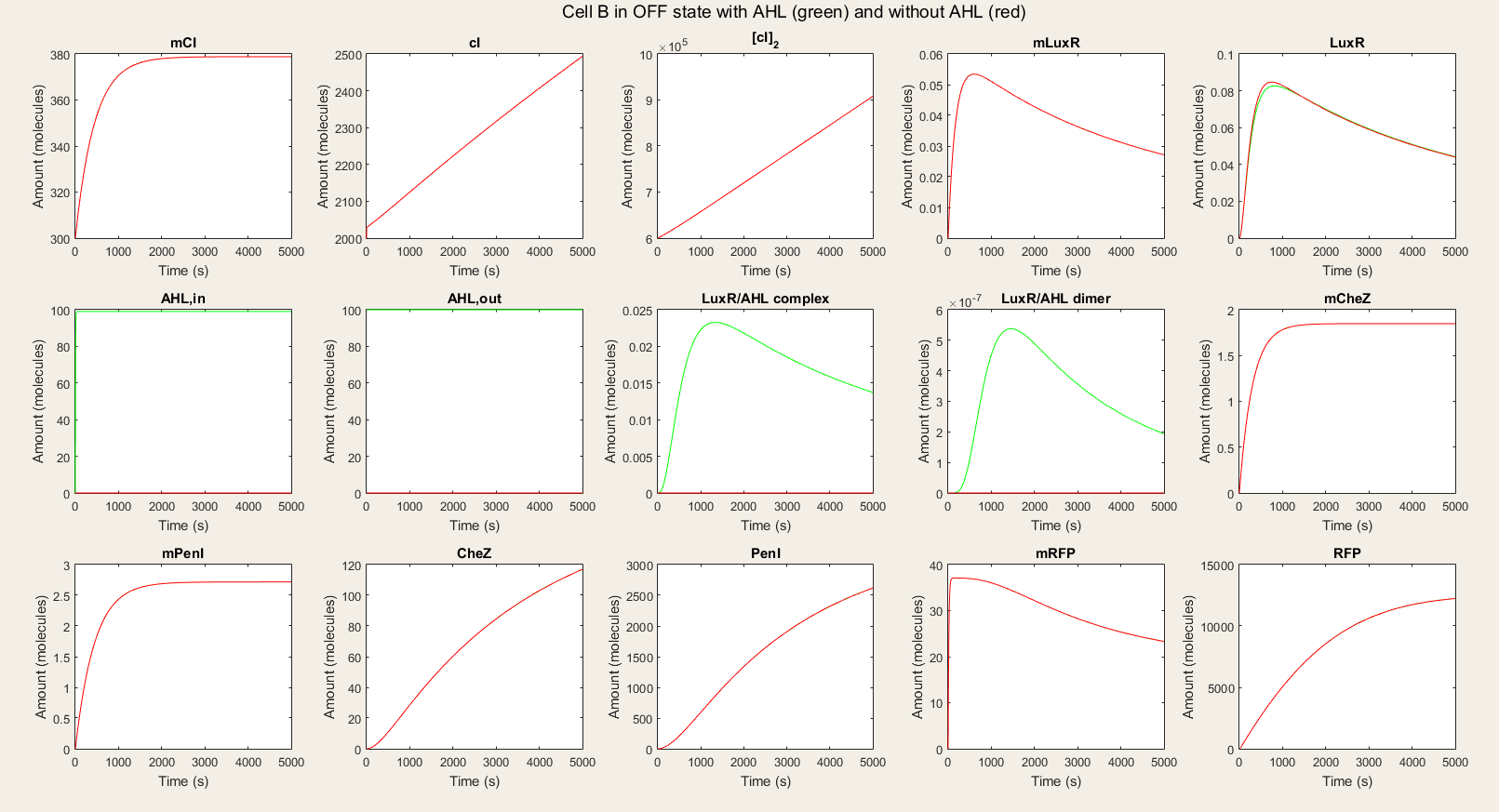#### Figure 4: Simulation of all processes in Cell B in OFF state with and without AHL induction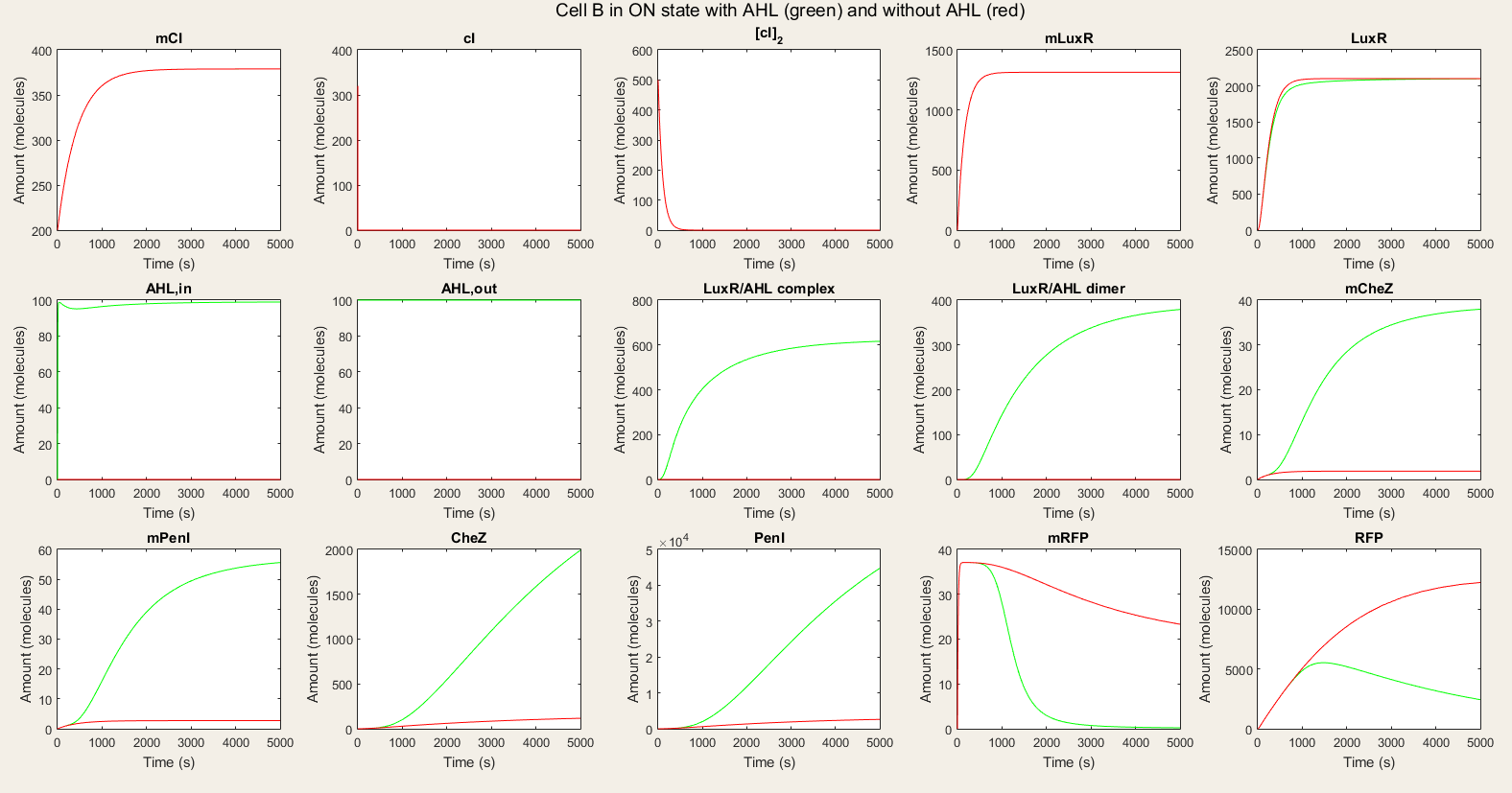#### Figure 5: Simulation of all processes in Cell B in ON state with and without AHL induction

It is also important to study the effect of change of AHL on cell B. Thus, we gave cell B a shot of AHL which is degraded in time and watched how it responded on it. Our simulation results were plotted in figure 6. We see that cell B starts with CheZ production untill a maximum, after which production slows down and the protein is degraded. This corresponds with a decrease in AHL concentration. After some time, cell B's internal network has switched and RFP production starts. We notice that the production rate is slower than CheZ production. From this simulation we can conclude that the network we modeled is able to adapt to the AHL concentration and that the reaction is the wanted reaction. We do also notice that it takes a long time (10 000 s) until most CheZ is degraded. This can form a barrier on the real life performance, since only a small amount of CheZ could suffice to give the cells the possibility to move.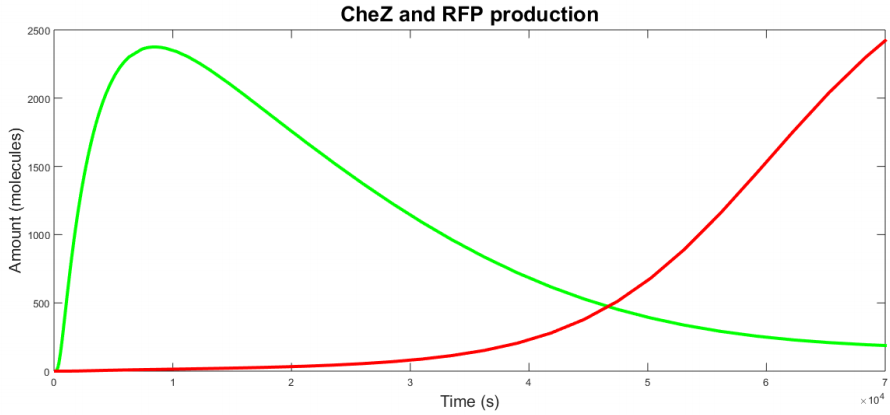#### Figure 6: Simulation of cell B with an initial shot of AHL

Sensitivity analysis

Now we are going to check which parameters have the biggest effect on the output and are the most important. We can quantify this effect using derivatives: $\frac{\delta {output}}{\delta {parameter}}$. The parameters with the largest sensitivity values, are the ones that should be best characterized. Furthermore, if they are controllable, they could be varied to our wishes. The sensitivity analysis will be executed in Simbiology. This analysis uses complex-step approximation to calculate derivatives of reaction rates. This technique yields accurate results for the vast majority of typical reaction kinetics including ours. We will use full dedimensionalization so we can compare the results.

For cell A the outputs are the amount of leucine and AHL in the medium and Adhesine. We took a time integral of the sensitivities and plotted it in figure 7.

The leucine medium is dependent on diffusion of leucine out, leucine consumption, degradation, transcription and translation of ilvE mRNA. We also notice that not all Ping-Pong Bi-Bi constants are equally important. The parameters kf1, kf-1 and kcat are the most important while kr1, kr-1 and kcat3 are the least important.

AHL medium is highly sensitive for variations in luxI translation, degradation of LuxI and LuxI mRNA and for the catalytic activity of LuxI.

From these results we can conclude that all outputs are dependent on variables closely related to themselves. Indeed, since there is production of AHL and LuxR in the cell, the transcriptional network is always active so the steps concerning the transcriptional network lose their importance. It is thus only a matter of understanding the metabolism, production and degradation terms to correctly model cell A.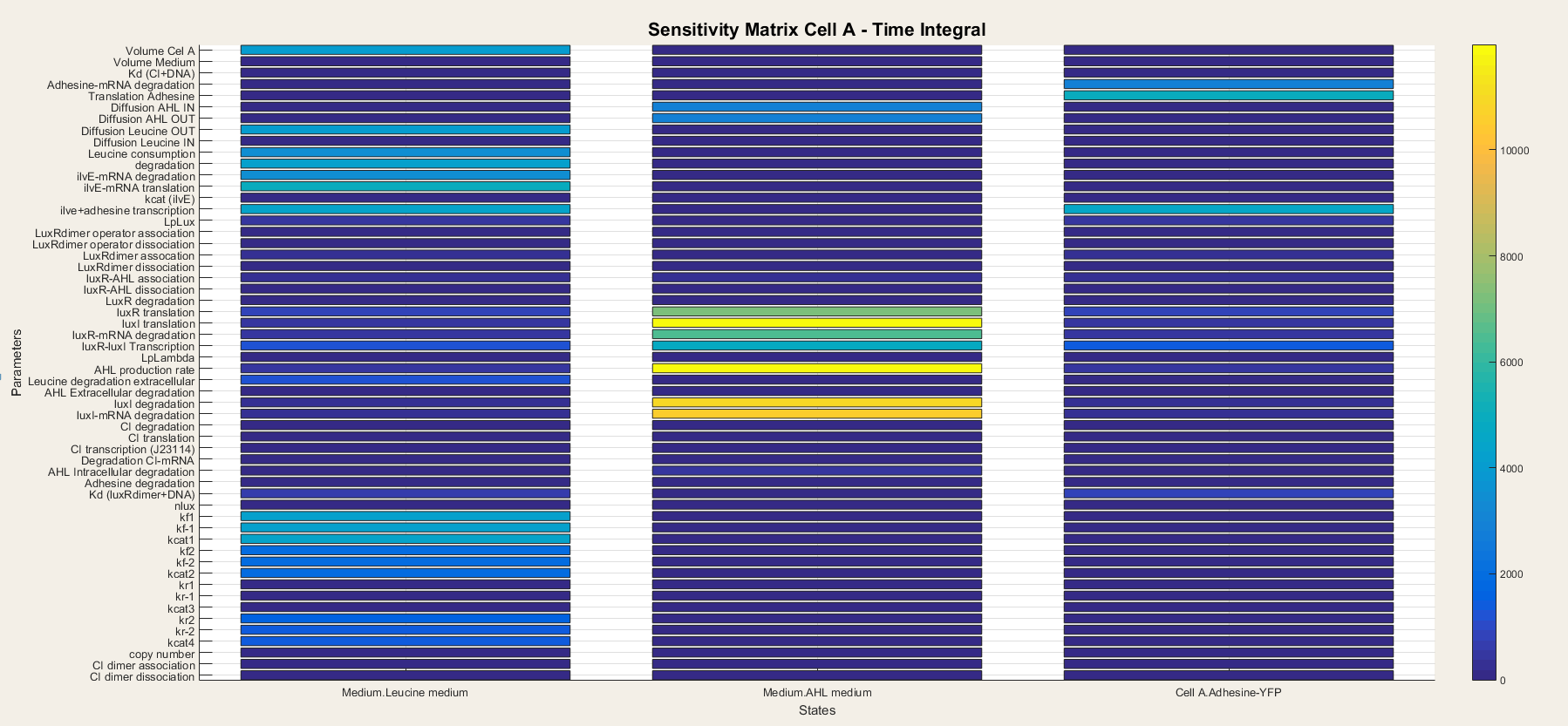#### Figure 7: Sensitivity analysis of parameters in cell A

We do the same for cell B. In cell B the output is the production of CheZ and RFP and the results are plotted in figure 8. We notice that CheZ-GFP is highly sensitive for the association rates of the LuxR/AHL complex and the LuxR/AHL dimer. RFP production is also sensitive for the association of the LuxR/AHL dimer.

We see that in cell B the dimerisation steps are really important. This is logical since cell B is dependent on external AHL concentrations to boot the transcriptional network. Thus, the steps concerning the binding of AHL to LuxR and making the activated LuxR/AHL dimer, which starts the transcription, are the most important steps. They determine the sensitivity of the network to AHL and as so, form the major component of the network.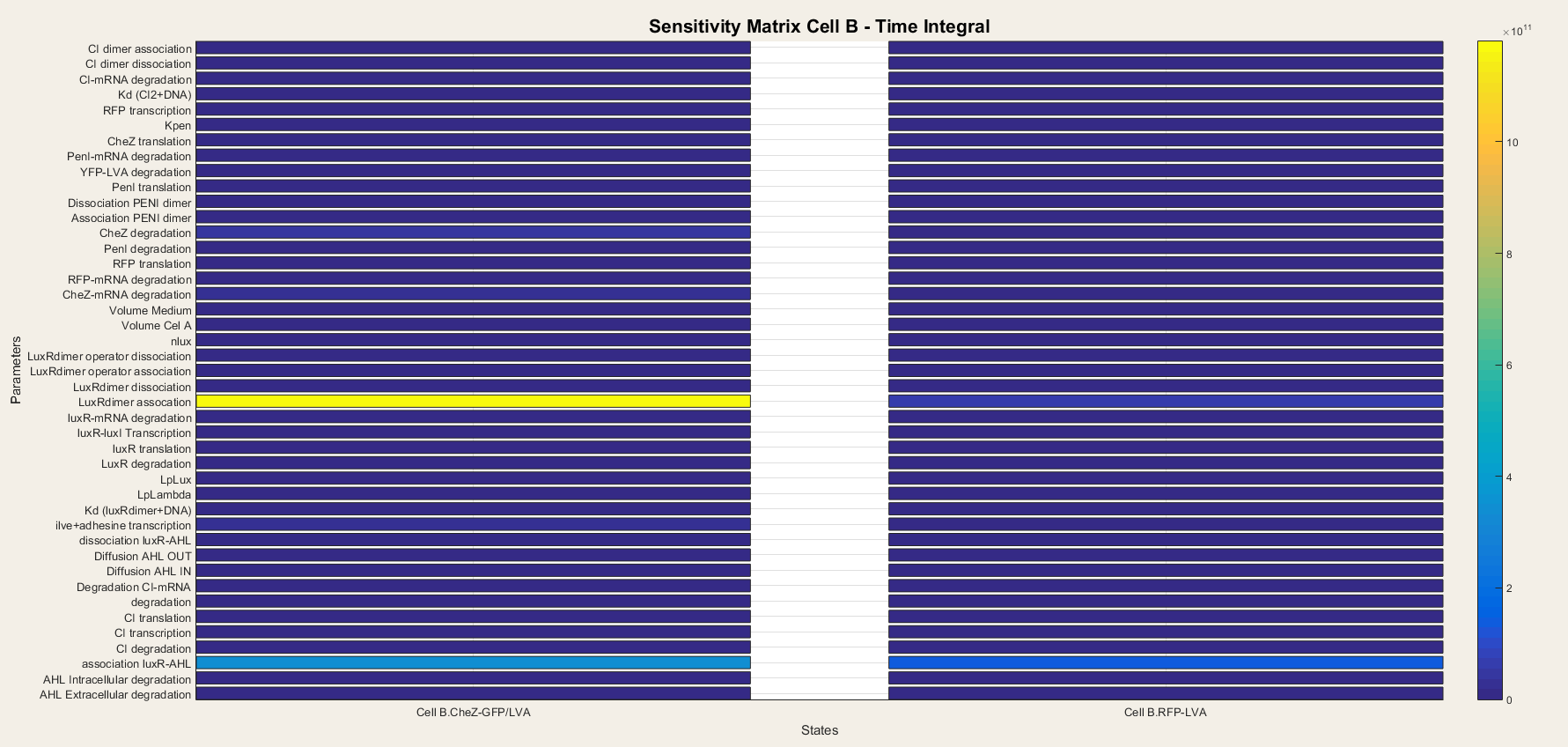#### Figure 8: Sensitivity analysis of parameters in cell B

Conclusion

We conclude from these results that our model displays the desired behavior. In both cell A and cell B, there is a big difference between cells in ON and OFF mode. In addition, the presence of AHL also has a big effect on cell B. The simulation also show a problem: altough we equiped the proteins with a LVA-tag, the degradation rate is still very slow and this can affect the cell behavior. We can also get semi-quantitative results from this model, but our assumption of an unlimited substrate pool leads to very high amount of biomolecules. A next step would be to fit some model parameters to wet lab data.

From the sensitivy analysis, we know which parameters are the most important and should be optimized. For cell A we should focus on metabolic, transcriptional and translational terms and for cell B we should focus on the dimerization steps.## References

  R. P. Shetty and B. Canton. PoPS. Openwetware 2007. [Available at url ]  Jason R Kelly, Adam J Rubin, Joseph H Davis, Caroline M Ajo-Franklin, John Cumbers, Michael J Czar, Kim de Mora, Aaron L Glieberman, Dileep D Monie, and Drew Endy. Measuring the activity of BioBrick promoters using an in vivo reference standard. Journal of biological engineering, 3:4, 2009. [ DOI ]  J N Weiss. The Hill equation revisited: uses and misuses. The FASEB journal : official publication of the Federation of American Societies for Experimental Biology, 11(11):835-841, 1997. [ DOI ]  Yufang Wang, Ling Guo, Ido Golding, Edward C Cox, and N P Ong. Quantitative transcription factor binding kinetics at the single-molecule level. Biophysical Journal, 96(2):609-620, 2009. [ DOI | arXiv | http ]  S. Basu, Y. Gerchman, C.H. Collins, F.H. Arnold and R. Weiss. A synthetic multicellular system for programmed pattern formation. Nature, 434(7037):1130-1134, 2005. [ DOI |  V Wittman, H C Lin, and H C Wong. Functional domains of the penicillinase repressor of Bacillus licheniformis. Journal of Bacteriology, 175(22):7383-7390, 1993.  Farasat I., Kushwaha M., Collens J., Easterbrook M. and H.M. Salis Efficient search, mapping, and optimization of multi-protein genetic systems in diverse bacteria. Molecular systems biology10(6):731, 1998. [ DOI ]  Benjamin Reeve, Thomas Hargest, Charlie Gilbert, and Tom Ellis. Predicting Translation Initiation Rates for Designing Synthetic Biology. Frontiers in Bioengineering and Biotechnology, 2(January):1-6, 2014. [ DOI | http ]  Howard M. Salis, Amin E. Borujeni and Anirudh S. Channarasappa. Translation rate is controlled by coupled trade-offs between site accessibility, selective RNA unfolding and sliding at upstream standby sites. Nucleic Acids Research 42 (2): 2646-2659, 2014. [ DOI |  A. B. Goryachev, D. J. Toh, and T. Lee. Systems analysis of a quorum sensing network: Design constraints imposed by the functional requirements, network topology and kinetic constants. In BioSystems, volume 83, pages 178-187, 2006. [ DOI ]  K T Samiee, M Foquet, L Guo, E C Cox, and H G Craighead. lambda-Repressor oligomerization kinetics at high concentrations using fluorescence correlation spectroscopy in zero-mode waveguides. Biophysical journal, 88(3):2145-2153, 2005. [ DOI | http ]  A L Schaefer, D L Val, B L Hanzelka, J E Cronan, and E P Greenberg. Generation of cell-to-cell signals in quorum sensing: acyl homoserine lactone synthase activity of a purified Vibrio fischeri LuxI protein. Proceedings of the National Academy of Sciences of the United States of America, 93(18):9505-9509, 1996. [ DOI ]  Chin-Rang Yang, Bruce E Shapiro, She-Pin Hung, Eric D Mjolsness, and G Wesley Hatfield. A mathematical model for the branched chain amino acid biosynthetic pathways of Escherichia coli K12. The Journal of biological chemistry, 280(12):11224-11232, 2005. [ DOI ]  Chin Rang Yang, Bruce E Shapiro, Eric D Mjolsness, and G Wesley Hatfield. An enzyme mechanism language for the mathematical modeling of metabolic pathways. Bioinformatics, 21(6):774-780, 2005. [ DOI ]  Jonathan a Bernstein, Arkady B Khodursky, Pei-Hsun Lin, Sue Lin-Chao, and Stanley N Cohen. Global analysis of mRNA decay and abundance in Escherichia coli at single-gene resolution using two-color fluorescent DNA microarrays. Proceedings of the National Academy of Sciences of the United States of America, 99(15):9697-9702, 2002. [ DOI ]  Jens Bo Andersen, Claus Sternberg, Lars Kongsbak Poulsen, Sara Petersen Bjø rn, Michael Givskov, and Sø ren Molin. New unstable variants of green fluorescent protein for studies of transient gene expression in bacteria. Applied and Environmental Microbiology, 64(6):2240-2246, 1998. [ arXiv ]  Horswill A.R., Stoodley P., Stewart P.S. and Parsek M.R. The effect of the chemical, biological, and physical environment on quorum sensing in structured microbial communities Anal Bioanal Chem.387(2):371-380.

### Contact

Address: Celestijnenlaan 200G room 00.08 - 3001 Heverlee
Telephone: +32(0)16 32 73 19
Email: igem@chem.kuleuven.be